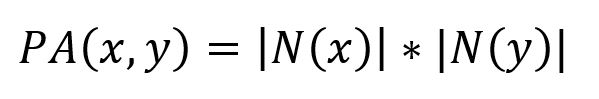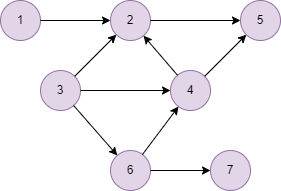# Change Nickname

Current Nickname:

Certifications

Certificate Issued at Valid until Serial No. File
Serial No. Valid until

Not having one? Apply now! >>>

Invoice

ProductName CreateTime ID Price File
ProductName CreateTime ID Price File

# Preferential Attachment

## Overview

Preferential attachment refers to a common phenomenon in complex network that the more connections a node has, the more likely it establishes new connections. If both nodes have a large number of connections, there is great possibility that they will be connected. In 2002, this method is used by A. Barabási and R. Albert in their proposed BA model for producing random scale-free networks:

## Basic Concept

### Preferential Attachment

Preferential attachment measurement uses the product of the number of neighbors of two nodes to determine their closeness, which is calculated by the following formula:where `N(x)` and `N(y)` are neighbor sets of `x` and `y` respectively. The larger the value of `PA(x,y)` is, the closer the two nodes are, value of 0 indicates that the two nodes are not close.Taking the above graph as an example, the blue node has 3 neighbors, the red node has 4 neighbors, so the score of their preferential attachment is `3 * 4 = 12`.

## Special Case

### Lonely Node, Disconnected Graph

Lonely node does not have any neighbor node, the algorithm does not calculate the Preferential Attachment between lonely node and any other node, either it considers the Preferential Attachment of two nodes which are located in different connected components.

### Self-loop Edge

The algorithm ignores all self-loop edges when calculating neighbor nodes.

### Directed Edge

For directed edges, the algorithm ignores the direction of edges but calculates them as undirected edges.

## Results and Statistics

Take the graph below as an example, run the algorithm in the graph:Algorithm results: Calculate the Preferential Attachment between node 3 and other nodes, return `node1`, `node2` and `num`

node1 node2 num
3 1 3
3 2 12
3 4 12
3 5 6
3 6 9
3 7 3

Algorithm statistics: N/A

## Command and Configuration

• Command: `algo(topological_link_prediction)`
• Configurations for the parameter `params()`:
Name
Type
Default
Specification Description
ids / uuids []`_id` / []`_uuid` / Mandatory IDs or UUIDs of the first set of nodes to be calculated, only need to configure one of them; every node in ids/uuids will be paired with every node in ids2/uuids2 for calculation
ids2 / uuids2 []`_id` / []`_uuid` / Mandatory IDs or UUIDs of the second set of nodes to be calculated, only need to configure one of them; every node in ids/uuids will be paired with every node in ids2/uuids2 for calculation
type string Adamic_Adar Adamic_Adar / Common_Neighbors / Preferential_Attachment / Resource_Allocation / Total_Neighbors Measurement of the closeness of the node pair; Adamic_Adar means to calculate AA index, Common_Neighbors means to calculate the number of common neighbors, Preferential_Attachment means to calculate the score of preferential attachment, Resource_Allocation means to calculate the score of resource allocation, Total_Neighbors means to calculate the number of total neighbors
limit int -1 >=-1 Number of results to return; return all results if sets to -1 or not set

## Algorithm Execution

#### 1. File Writeback

Configuration Data in Each Row
filename `node1`,`node2`,`num`

Example: Calculate the Preferential Attachment of node UUID = 3 and all other nodes, write the algorithm results back to file named pa

``````algo(topological_link_prediction).params({
uuids: ,
uuids2: [1,2,4,5,6,7],
type: "Preferential_Attachment"
}).write({
file:{
filename: "pa"
}
})
``````

#### 2. Property Writeback

Not supported by this algorithm.

#### 3. Statistics Writeback

This algorithm has no statistics.

### Direct Return

Alias Ordinal Type
Description
Column Name
0 []perNodePair Closeness of node pair `node1`, `node2`, `num`

Example: Calculate the Preferential Attachment of node UUID = 3 and UUID = 4, define algorithm results as alias named pa and return the results

``````algo(topological_link_prediction).params({
uuids: ,
uuids2: ,
type: "Preferential_Attachment"
}) as pa
return pa
``````

### Streaming Return

Alias Ordinal Type
Description
Column Name
0 []perNodePair Closeness of node pair `node1`, `node2`, `num`

Example: Calculate the Preferential Attachment of node UUID = 1 and UUID = 5,6,7, return the results in the descending closeness score

``````algo(topological_link_prediction).params({
uuids: ,
uuids2: [5,6,7],
type: "Preferential_Attachment"
}).stream() as pa
return pa order by pa.num desc
``````

### Real-time Statistics

This algorithm has no statistics.